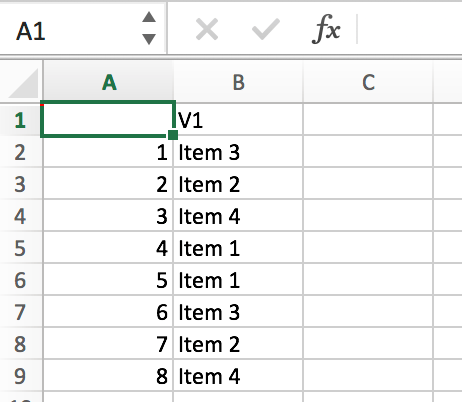# Create a random list generator in R that runs multiple times

We are often showing participants different stimuli that are randomly ordered to prevent ordering effects. To randomize the stimuli we often used random.org’s list randomizer, but a current limitation of their site is you can only randomize a list once. So, for instance, if we were testing 4 stimuli with four people, we would need to use random.org to randomize that list four different times.

Here is some simple code to create a random list generator that will resample a list as many times as you want. You enter the number of items in your list, the number of times you want to draw down your entire list, and define the items in your list. The code below puts the list items into a single column and then prints the results to a CSV file. The example code has 4 items in the list and will randomize those 4 items 2 separate times. Sampling is done without replacement.

```# Enter the number of items in your list
x <- 4

#Enter the number of times you want the list to run
r <- 2

#Add the items that are in your list. Add to or remove the number of items if necessary
mylist <- c('Item 1','Item 2','Item 3','Item 4')

#Below is the randomizer
randommatrix <- matrix(nrow=x,ncol=r)
for (i in 1:r)
{randommatrix[,i]=sample(mylist,x,replace=FALSE)}
y <- x*r
randommatrixlist <- matrix(randommatrix,nrow=y,ncol=1)
print(randommatrixlist)

#Optional: Print your randomization list to a CSV file. Delete the "#" to activate
write.csv(randommatrixlist,'randomlist.csv')
```

This is the result, though obviously this list will likely look different when you run the code.
``` [1,] "Item 3" [2,] "Item 2" [3,] "Item 4" [4,] "Item 1" [5,] "Item 1" [6,] "Item 3" [7,] "Item 2" [8,] "Item 4" ```

Here is what the resulting CSV file looks like in Excel.Tags